# Trajectory of a Particle in a Magnetic Field

Here is a problem shared with me.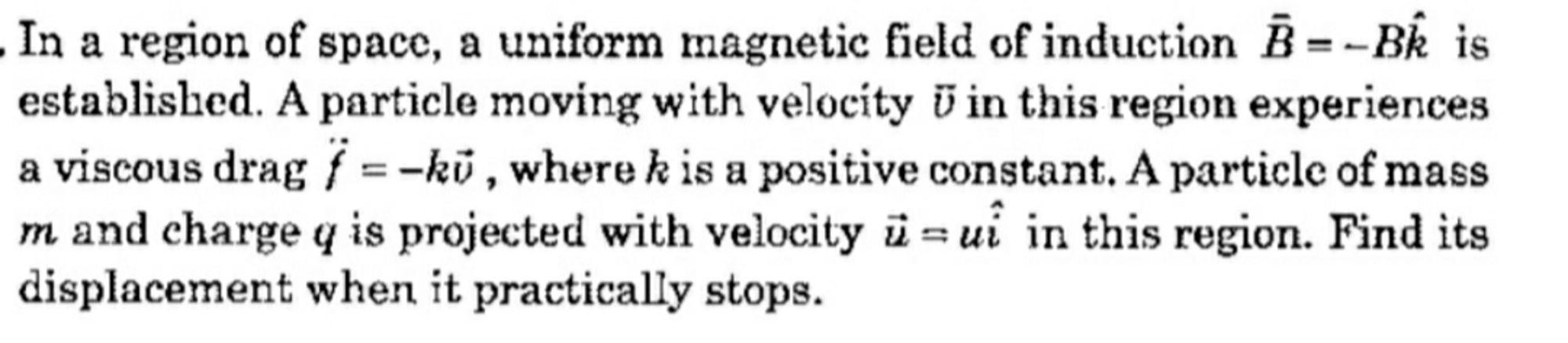At any instant of time, let the particle's position and velocity be:

$\vec{r} = x \ \hat{i} + y \ \hat{j}$ $\vec{v} = v_x \ \hat{i} + v_y \ \hat{j}$

Applying Newton's second law at this instant:

$m\vec{a} =m(\dot{v}_x \ \hat{i} + \dot{v}_y \ \hat{j})= q(\vec{v}\times \vec{B}) - k \vec{v}$

Simplifying gives:

$m\dot{v}_x = -qBv_y -kv_x \ \dots(1)$ $m\dot{v}_y = qBv_x-kv_y \ \dots(2)$

Let:

$a = \frac{k}{m}$ $b = \frac{qB}{m}$

The differential equations become:

$\dot{v}_x = -av_x -bv_y \ \dots(3)$ $\dot{v}_y = bv_x - av_y \ \dots(4)$

$v_x(0)=u \ ; \ v_y(0) = 0$

Differentiating (3) wrt. time:

$\ddot{v}_x = -a\dot{v}_x -b\dot{v}_y$

Plugging in $\dot{v}_y$ above gives: $\implies \ddot{v}_x = -a\dot{v}_x - b (bv_x - av_y )$ $\implies \ddot{v}_x = -a\dot{v}_x - b^2 v_x + abv_y \ \dots(5)$

Again, looking at (3):

$bv_y = - \dot{v}_x - av_x$ Plugging the above in (5) and simplifying gives:

$\ddot{v}_x + 2a\dot{v}_x + (a^2+b^2)v_x=0$

A similar operation can be performed and the following equation can be obtained: $\ddot{v}_y + 2a\dot{v}_y + (a^2+b^2)v_y=0$

$v_x(0)=u \ ; \ \dot{v}_x(0) = -au \ ; \ v_y(0)=0 \ ; \ \dot{v}_y(0) = bu$

Let:

$2a = p$ $a^2 + b^2 = q$

This implies:

$\ddot{v}_x + p\dot{v}_x + qv_x=0$

The roots of the above characteristic equation are:

$k_1 = \frac{-p+\sqrt{p^2-4q}}{2} = -a + ib$ $k_2 = \frac{-p-\sqrt{p^2-4q}}{2}=-a-ib$

Where $i= \sqrt{-1}$

$v_x = \mathrm{e}^{-at} \left(c_1 \sin{bt} + c_2 \cos{bt}\right)$ $v_y = \mathrm{e}^{-at} \left(c_3 \sin{bt} + c_4 \cos{bt}\right)$

The above constants $c_{1,2,3,4}$ can be obtained by applying initial conditions.

Know that:

$x(0) = y(0) =0$

Integrating the velocities will give the displacements along each direction. It can be seen that as time increases, the particle eventually comes to rest. So the displacement when the particle comes to rest can be found as such:

$x_D = \lim_{t \to \infty} x(t)$ $y_D = \lim_{t \to \infty} y(t)$

$D = \sqrt{x_D^2 + y_D^2}$

I have not done all the calculations as doing so will take me time. I have laid out the steps to solve this problem.

Edit: I have solved for the particle's motion numerically with the following parameters:

$B = q=m = u=1$

The trajectory is plotted for various $k$ as follows. One can see that when $k=0$ one gets the classical circular motion result. For the other cases, the motion is a stable spiral trajectory.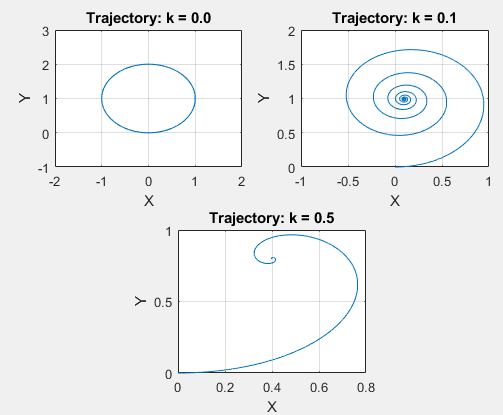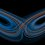Note by Karan Chatrath
1 month, 2 weeks ago

This discussion board is a place to discuss our Daily Challenges and the math and science related to those challenges. Explanations are more than just a solution — they should explain the steps and thinking strategies that you used to obtain the solution. Comments should further the discussion of math and science.

When posting on Brilliant:

• Use the emojis to react to an explanation, whether you're congratulating a job well done , or just really confused .
• Ask specific questions about the challenge or the steps in somebody's explanation. Well-posed questions can add a lot to the discussion, but posting "I don't understand!" doesn't help anyone.
• Try to contribute something new to the discussion, whether it is an extension, generalization or other idea related to the challenge.

MarkdownAppears as
*italics* or _italics_ italics
**bold** or __bold__ bold
- bulleted- list
• bulleted
• list
1. numbered2. list
1. numbered
2. list
Note: you must add a full line of space before and after lists for them to show up correctly
paragraph 1paragraph 2

paragraph 1

paragraph 2

[example link](https://brilliant.org)example link
> This is a quote
This is a quote
    # I indented these lines
# 4 spaces, and now they show
# up as a code block.

print "hello world"
# I indented these lines
# 4 spaces, and now they show
# up as a code block.

print "hello world"
MathAppears as
Remember to wrap math in $$ ... $$ or $ ... $ to ensure proper formatting.
2 \times 3 $2 \times 3$
2^{34} $2^{34}$
a_{i-1} $a_{i-1}$
\frac{2}{3} $\frac{2}{3}$
\sqrt{2} $\sqrt{2}$
\sum_{i=1}^3 $\sum_{i=1}^3$
\sin \theta $\sin \theta$
\boxed{123} $\boxed{123}$

Sort by:

@Karan Chatrath the question in the book is asking for (velocity of the particle A when angle between the rods becomes $\theta$)
Can you show your attempt by posting a discussion ?

- 1 month ago

I will create a note after some time.

- 1 month ago

Which question? I would use Lagrangian mechanics.

- 1 month ago

Mechanics |17-09-2020|

- 1 month ago

I'm trying it out right now. I'm trying to create a time-domain simulation.

- 1 month ago

@Karan Chatrath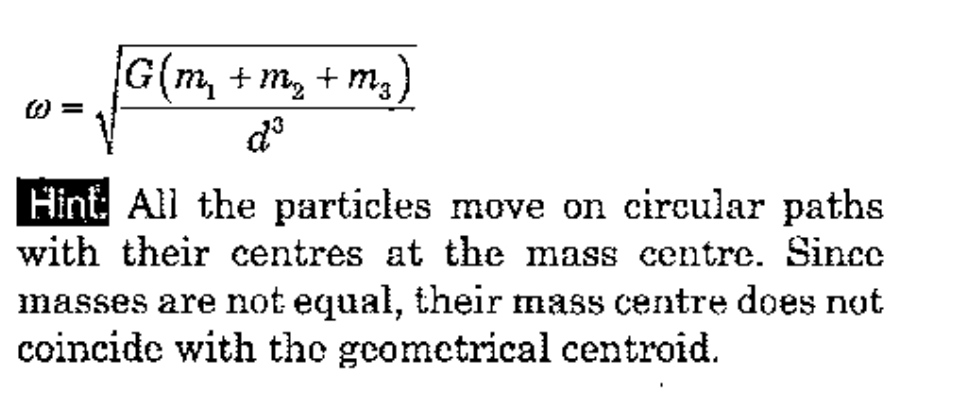Sir i want to see your solution. Share whenever you will be free.

- 1 month ago

@Karan Chatrath at $t=\frac{mπ}{qB}$ the velocity vector turns by $2π$
I can find the displacement from origin but cannot able to find distance. The particle has a weird trajectory, how to calculate distance travelled , Give me some clue /hint?

- 1 month, 1 week ago

No, the time you have computed is incorrect.

For distance:

$ds = V \ dt$ Where $V$ is the speed.

- 1 month, 1 week ago

@Karan Chatrath the attempt is posted.

- 1 month, 1 week ago

@Karan Chatrath after 20 minutes I am going to post a note sharing my attempt Please be active after 20 minutes.

- 1 month, 1 week ago

@Karan Chatrath sir if you don't mind, there is an error in 15th line of solution it should be $a^{2}+b^{2}$

- 1 month, 1 week ago

Good catch. It is corrected now.

- 1 month, 1 week ago

@Steven Chase @Karan Chatrath @Krishna Karthik i have changed the graph little bit and added Note on the problem spaceship Mechanics.
I hope the problem is clearly stated now.

- 1 month, 1 week ago

I still don't understand. Finding the time as a function of initial time is hard, but necessary. It must be done in order to achieve a final expression!

- 1 month, 1 week ago

His answer is around $0.053$ ?

- 1 month, 1 week ago

@Steven Chase @Karan Chatrath I have posted the problem again with right answer here

- 1 month, 1 week ago

@Karan Chatrath i have made necessary changes in the spaceship Mechanics.
And within some hours I will post a note showing my attempt on that charge problem.

- 1 month, 1 week ago

I have tried that problem and I do not know how to solve it. In my opinion, the available information is inadequate, but I may be wrong.

- 1 month, 1 week ago

I agree as well. I think the question should tell what the final time is, at least. There's no way there's enough information. The most I got up to was this:

The velocity of the rocket when it is switched of can be given by:

$\displaystyle v_0 = \sqrt{\frac{25}{12} GM \left(\frac{R-R_0}{R_0 R} \right) }$

I solved it by separating and integrating:

$\displaystyle \int_{v_0}^{\frac{1}{5}v_0} v dv = \int_{R_0}^{R} -\frac{GM}{R^2} dR$

But the question is: how do I find the final distance, when the rocket reaches constant velocity?

Edit: I managed to write the final distance as a function of time when the rocket reaches a fifth of its initial velocity. But if you aren't given the anything else, the question is impossible to solve.

Would it be right in saying that the distance from Earth as a function of time would be $\displaystyle R = e^{\sqrt{-2GM}t}$?

The golden question is: How is the final time related to $t_0$?

- 1 month, 1 week ago

I thought the problem was telling us that $5 t_0$ was the time it took for the velocity to drop to $e^{-5} v_0$. Like a "five time constant" thing. Which doesn't quite make sense, because the solution to the diff eq shouldn't be an exponential. Anyway, it rejected my answer too (I tried typing in 0.053)

- 1 month, 1 week ago

@Krishna Karthik where did karan sir gone.
He was talking with us

- 1 month, 1 week ago

Let him be in peace. He should focus in order to solve the problem.

- 1 month, 1 week ago

@Krishna Karthik Hope @Karan Chatrath will post a solution of Spaceship Mechanics

- 1 month, 1 week ago

@Lil Doug I really do. That one baffles the shit out of me. Btw did you see my approach on it?

The velocity of the rocket when it is switched of can be given by:

$\displaystyle v_0 = \sqrt{\frac{25}{12} GM \left(\frac{R-R_0}{R_0 R} \right) }$

I solved it by separating and integrating:

$\displaystyle \int_{v_0}^{\frac{1}{5}v_0} v dv = \int_{R_0}^{R} -\frac{GM}{R^2} dR$

But the question is: how do I find the final distance, when the rocket reaches constant velocity?

- 1 month, 1 week ago

@Krishna Karthik Bro let me try again the problem.

- 1 month, 1 week ago

Is my approach correct? Btw did you also start with this? Also, you can do this by linking using the work energy theorem (no differential equation solving there):

$\displaystyle U_i = \frac{-GMm}{R}$

$\displaystyle U_f = \frac{-GMm}{R_0}$

$\displaystyle \frac{1}{50}mv_0^2 - \frac{1}{2}mv_0^2 = U_f - U_i$

You can get the exact same result with the above method.

- 1 month, 1 week ago

@Krishna Karthik I don't know because I didn't have tried the problem with even $50\%$ of my full potential

I was feeling lazy, just posted it bro.

- 1 month, 1 week ago

No worries my dude. This kind of problem; yikes. People in SpaceX working for Elon Musk be trynna solve this shit...

- 1 month, 1 week ago

@Krishna Karthik is Elon Musk sir is in Brilliant.?

- 1 month, 1 week ago

Lol nope. Yeah; people there would struggle solving this problem. SpaceX is extremely good with their rockets.

- 1 month, 1 week ago

@Krishna Karthik what is the meaning of “people there would struggle solving this problem.“
I don't think people of spacex will difficulty to solve that problem.

- 1 month, 1 week ago

Not really, but it's still super hard to do by pencil and paper. Besides, real rocket engineers probably haven't tried a problem under exam conditions in ages.

But this is a really tough one with lots of mathematically hard stuff. If you can somehow find Stephen Hawking in heaven and bring him back to Earth, he'll do it for you :)

- 1 month, 1 week ago

@Krishna Karthik I agree.
Now I have some work, I am going, we will talk tomorrow regarding rocket problem .
By the way within 20min a new song of my favorite singer is coming, so I am very excited.
Bye.

- 1 month, 1 week ago

Ok bud. See ya. I've gotta go to sleep anyway.

- 1 month, 1 week ago

@Krishna Karthik it seems me that you are very interested in rocket.

- 1 month, 1 week ago

It's an interesting question.

- 1 month, 1 week ago

@Krishna Karthik @Karan Chatrath I have made changes in the spaceship mechanics problem, the time at which the rocket turned off is $t_{0}$

- 1 month, 1 week ago

Please tell me. How do I do the rocket problem?

- 1 month, 1 week ago

Hi Krishna, please refrain from using swear words on Brilliant. I've updated your comment.

Staff - 1 month, 1 week ago

@Krishna Karthik I have also posted for solution 😜
I was also not able to solve that.

- 1 month, 1 week ago

Here's what I got up to:

The velocity of the rocket when it is switched of can be given by:

$\displaystyle v_0 = \sqrt{\frac{25}{12} GM \left(\frac{R-R_0}{R_0 R} \right) }$

I solved it by separating and integrating:

$\displaystyle \int_{v_0}^{\frac{1}{5}v_0} v dv = \int_{R_0}^{R} -\frac{GM}{R^2} dR$

But the question is: how do I find the final distance, when the rocket reaches constant velocity?

- 1 month, 1 week ago

@Karan Chatrath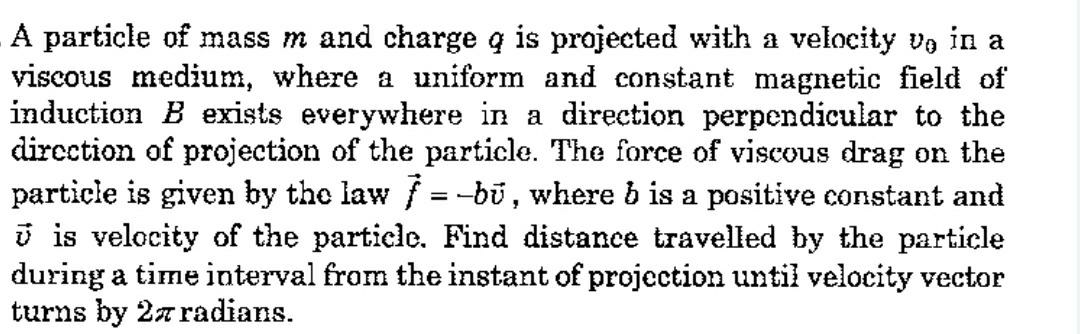- 1 month, 1 week ago

$D = \frac{mv_o}{b}\left(1 - \mathrm{e}^{-\frac{2 \pi b}{qB}}\right)$

- 1 month, 1 week ago

@Karan Chatrath yes it's $\Huge CORRECT$

- 1 month, 1 week ago

@Lil Doug Lol that's a funny way of saying correct. lmao. You gave me a fright.🤣

- 1 month, 1 week ago

@Krishna Karthik he is my favorite teacher.
He is just awesome bro.
Therefore I done that. His skills of solving problem are better than even Einstein sir.

- 1 month, 1 week ago

What set Einstein apart was creativity, not problem-solving. But, both do go hand in hand in some way. He is quite remarkable, Karan Chatrath.

- 1 month, 1 week ago

I will try it, but I want to see your attempt before I post mine. Also, I posted a report on your problem titled 'Spaceship Mechanics'. Could you please get back to me on that?

- 1 month, 1 week ago

About your report, $t_0$ is when the engine is turned off, I think.

- 1 month, 1 week ago

@Karan Chatrath Yes sir, sorry I didn't reply you for that report.
The question is basically copied through the book, I checked it many times, there is nothing more than that given in question.
Can you please try to think it by seeing the graph.

- 1 month, 1 week ago

$t_0$ I think is the time when the engine is turned off

- 1 month, 1 week ago

@Karan Chatrath Try this problem.

- 1 month, 1 week ago

@Lil Doug By the way, this is the same problem as the damping one you wanted me to solve, just with a little different final quantity to be solved for. Latex is being very buggy in the notes section, and I just lost a bunch of work trying to post the solution.

- 1 month, 1 week ago

@Steven Chase I am not able to understand only that last line (velocity vector turns my $2π$
Can you help me to understand that line.
Hope I am not disturbing you.

- 1 month, 1 week ago

The motion is described in terms of sinusoids of a certain frequency. You can find out what the time period is for the sinusoids, and evaluate the motion up until one time period has elapsed.

- 1 month, 1 week ago

@Steven Chase sir i think the problem is whole same, except last line.
I have provided $x(t)$ and $y(t)$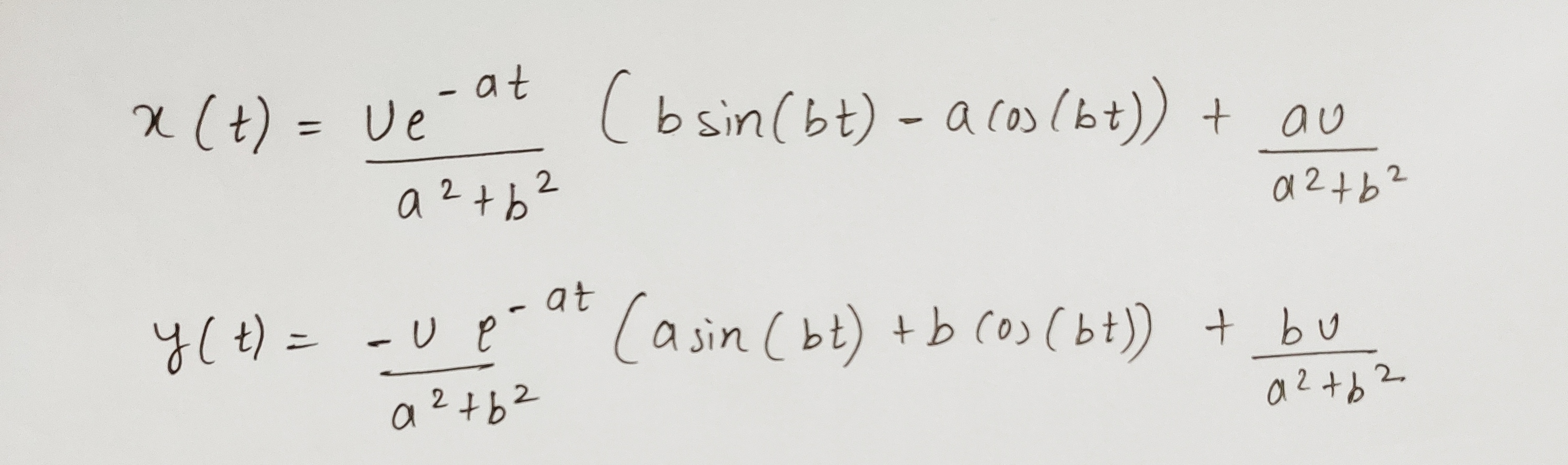Can you do something from this data? Thanks in advance. Hope I am not disturbing you

- 1 month, 1 week ago

@Steven Chase No problem :)

- 1 month, 1 week ago

@Karan Chatrath Yeah it worked finally.
The difference between your and my method is that , by your method we can find location of particle at any time t, and in my method I have just focused on where the particle stops.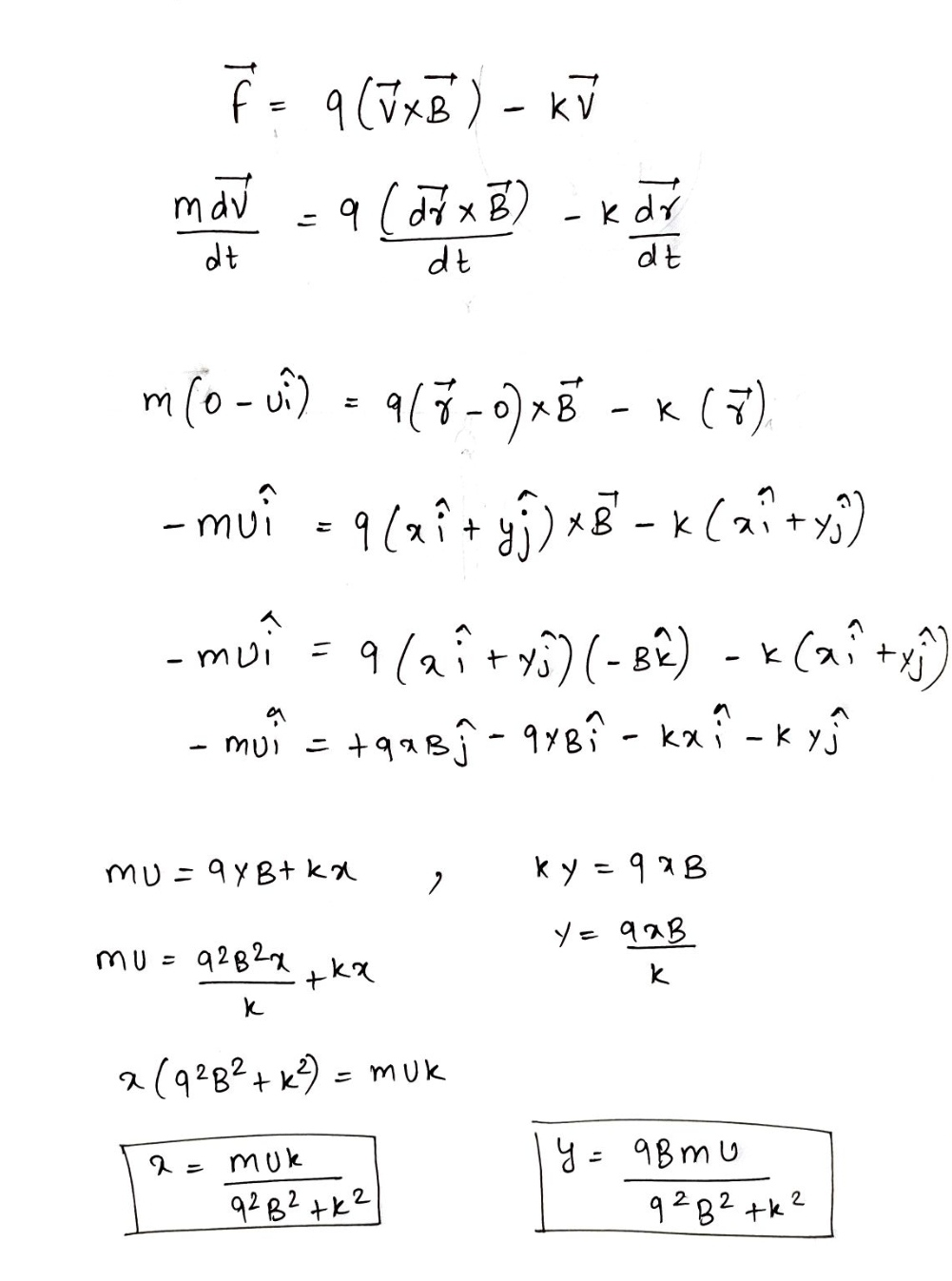Reply if you found it good.

- 1 month, 1 week ago

Yes, this is a faster way of solving the problem. Well done.

- 1 month, 1 week ago

I was looking at phase portraits earlier; thanks for this one!

- 1 month, 2 weeks ago

The plots that I have shared are not phase portraits.

- 1 month, 2 weeks ago

Yeah; I know that; these are trajectories, but they look like phase portraits of pendulums with drag.

- 1 month, 1 week ago

@Karan Chatrath and yes the answer is $\Large \vec{D}=\frac{kmu}{q^{2}B^{2}+k^{2}} \vec{i}+ \frac{qBmu}{ q^{2}B^{2}+k^{2} } \vec{j}$
Greetings we just rocked!
But your contribution is 95% :)

- 1 month, 2 weeks ago

Well done! Glad that I could help

- 1 month, 2 weeks ago

@Karan Chatrath You help always. Love you sir .
But this was very difficult, what do you think.

- 1 month, 2 weeks ago

Solving by hand makes this a difficult problem. It is easy to make calculation mistakes

- 1 month, 2 weeks ago

@Karan Chatrath after that- 1 month, 2 weeks ago

Based on this, as $t \to \infty$:

$x_D = \frac{au}{a^2 + b^2}$ $y_D = \frac{bu}{a^2 + b^2}$

- 1 month, 2 weeks ago

@Karan Chatrath After doing calculations I am getting $c_{1}=0$ $c_{2}=u$ $c_{3}=u$ $c_{4}=0$

- 1 month, 2 weeks ago

I have not checked this myself. But from a dimensional standpoint, the result seems okay.

I have edited the note by adding some plots. Hope this helps.

- 1 month, 2 weeks ago

@Karan Chatrath if you don't mind,if it takes very much time. To write all thing in latex, which maybe a waste of time, then I suggest you to use pen and page.

- 1 month, 2 weeks ago

@Karan Chatrath That really interesting.
I wish I could see particle whole motion in graph.

- 1 month, 2 weeks ago

I will update this note after some time. But if you put $k=0 \implies a=0$ the particle moves in a circle as expected.

- 1 month, 2 weeks ago

@Karan Chatrath acha to fir magnetic force aur drag force barabar ho jaayega to particle ruk jayega .
Right?, aisa aisa hua to bahut interesting ho jaayega

- 1 month, 2 weeks ago

@Karan Chatrath after integration of velocity , 2 more constants are waiting us.

- 1 month, 2 weeks ago

$x(0) = y(0) =0$

You missed this line too, so I am adding it here. Use these two conditions to solve for the constants.

- 1 month, 2 weeks ago

@Karan Chatrath Using two initial conditions , how can we find $4$ constants?

- 1 month, 2 weeks ago

$v_x(0)=u \ ; \ \dot{v}_x(0) = -Au \ ; \ v_y(0)=0 \ ; \ \dot{v}_y(0) = Bu$

You missed the above line in the note, so I am adding it here too.

- 1 month, 2 weeks ago

@Karan Chatrath oh yess sorry
If you don't mind we should not write it as B, because there are two B which leads to confusion.

- 1 month, 2 weeks ago

That is a good point. I have edited the note

- 1 month, 2 weeks ago

@Karan Chatrath will the particle come to at the rest after $t=\infty$ ?

- 1 month, 2 weeks ago

Yes, the particle will come to rest as $t \to \infty$ since the expression for the velocity components has a decaying exponent.

- 1 month, 2 weeks ago